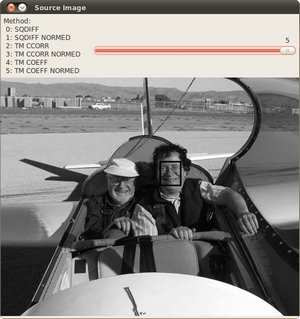OpenCV  3.4.15-dev Open Source Computer Vision
Template Matching

Prev Tutorial: Back Projection

Next Tutorial: Finding contours in your image

## Goal

In this tutorial you will learn how to:

• Use the OpenCV function matchTemplate() to search for matches between an image patch and an input image
• Use the OpenCV function minMaxLoc() to find the maximum and minimum values (as well as their positions) in a given array.

## Theory

### What is template matching?

Template matching is a technique for finding areas of an image that match (are similar) to a template image (patch).

While the patch must be a rectangle it may be that not all of the rectangle is relevant. In such a case, a mask can be used to isolate the portion of the patch that should be used to find the match.

### How does it work?

• We need two primary components:

1. Source image (I): The image in which we expect to find a match to the template image
2. Template image (T): The patch image which will be compared to the source image

our goal is to detect the highest matching area: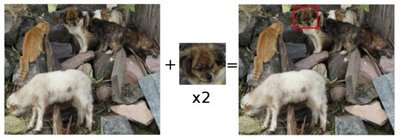• To identify the matching area, we have to compare the template image against the source image by sliding it: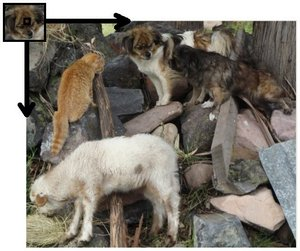• By sliding, we mean moving the patch one pixel at a time (left to right, up to down). At each location, a metric is calculated so it represents how "good" or "bad" the match at that location is (or how similar the patch is to that particular area of the source image).
• For each location of T over I, you store the metric in the result matrix R. Each location $$(x,y)$$ in R contains the match metric: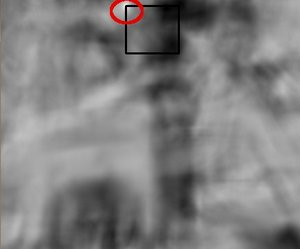the image above is the result R of sliding the patch with a metric TM_CCORR_NORMED. The brightest locations indicate the highest matches. As you can see, the location marked by the red circle is probably the one with the highest value, so that location (the rectangle formed by that point as a corner and width and height equal to the patch image) is considered the match.

• In practice, we locate the highest value (or lower, depending of the type of matching method) in the R matrix, using the function minMaxLoc()

### How does the mask work?

• If masking is needed for the match, three components are required:
1. Source image (I): The image in which we expect to find a match to the template image
2. Template image (T): The patch image which will be compared to the source image
• Only two matching methods currently accept a mask: TM_SQDIFF and TM_CCORR_NORMED (see below for explanation of all the matching methods available in opencv).
• The mask must have the same dimensions as the template
• The mask should have a CV_8U or CV_32F depth and the same number of channels as the template image. In CV_8U case, the mask values are treated as binary, i.e. zero and non-zero. In CV_32F case, the values should fall into [0..1] range and the template pixels will be multiplied by the corresponding mask pixel values. Since the input images in the sample have the CV_8UC3 type, the mask is also read as color image.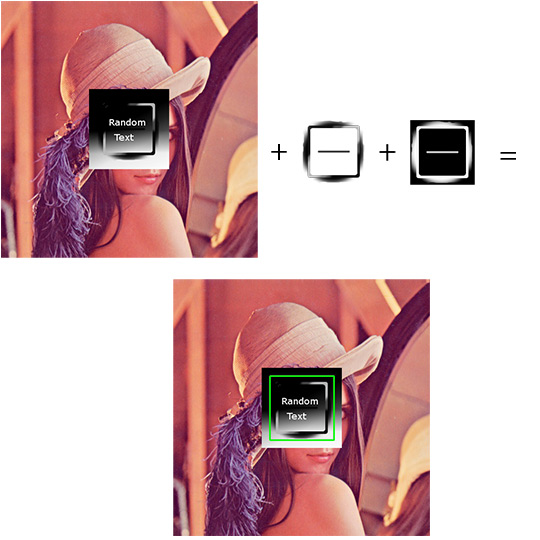### Which are the matching methods available in OpenCV?

Good question. OpenCV implements Template matching in the function matchTemplate(). The available methods are 6:

1. method=TM_SQDIFF

$R(x,y)= \sum _{x',y'} (T(x',y')-I(x+x',y+y'))^2$

2. method=TM_SQDIFF_NORMED

$R(x,y)= \frac{\sum_{x',y'} (T(x',y')-I(x+x',y+y'))^2}{\sqrt{\sum_{x',y'}T(x',y')^2 \cdot \sum_{x',y'} I(x+x',y+y')^2}}$

3. method=TM_CCORR

$R(x,y)= \sum _{x',y'} (T(x',y') \cdot I(x+x',y+y'))$

4. method=TM_CCORR_NORMED

$R(x,y)= \frac{\sum_{x',y'} (T(x',y') \cdot I(x+x',y+y'))}{\sqrt{\sum_{x',y'}T(x',y')^2 \cdot \sum_{x',y'} I(x+x',y+y')^2}}$

5. method=TM_CCOEFF

$R(x,y)= \sum _{x',y'} (T'(x',y') \cdot I'(x+x',y+y'))$

where

$\begin{array}{l} T'(x',y')=T(x',y') - 1/(w \cdot h) \cdot \sum _{x'',y''} T(x'',y'') \\ I'(x+x',y+y')=I(x+x',y+y') - 1/(w \cdot h) \cdot \sum _{x'',y''} I(x+x'',y+y'') \end{array}$

6. method=TM_CCOEFF_NORMED

$R(x,y)= \frac{ \sum_{x',y'} (T'(x',y') \cdot I'(x+x',y+y')) }{ \sqrt{\sum_{x',y'}T'(x',y')^2 \cdot \sum_{x',y'} I'(x+x',y+y')^2} }$

## Code

• What does this program do?
• Loads an input image, an image patch (template), and optionally a mask
• Perform a template matching procedure by using the OpenCV function matchTemplate() with any of the 6 matching methods described before. The user can choose the method by entering its selection in the Trackbar. If a mask is supplied, it will only be used for the methods that support masking
• Normalize the output of the matching procedure
• Localize the location with higher matching probability
• Draw a rectangle around the area corresponding to the highest match

## Explanation

• Declare some global variables, such as the image, template and result matrices, as well as the match method and the window names:
• Load the source image, template, and optionally, if supported for the matching method, a mask:
• Create the Trackbar to enter the kind of matching method to be used. When a change is detected the callback function is called.
• Let's check out the callback function. First, it makes a copy of the source image:
• Perform the template matching operation. The arguments are naturally the input image I, the template T, the result R and the match_method (given by the Trackbar), and optionally the mask image M.
• We normalize the results:
• We localize the minimum and maximum values in the result matrix R by using minMaxLoc().
• For the first two methods ( TM_SQDIFF and MT_SQDIFF_NORMED ) the best match are the lowest values. For all the others, higher values represent better matches. So, we save the corresponding value in the matchLoc variable:
• Display the source image and the result matrix. Draw a rectangle around the highest possible matching area:

## Results

1. Testing our program with an input image such as: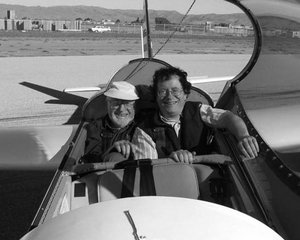and a template image: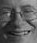2. Generate the following result matrices (first row are the standard methods SQDIFF, CCORR and CCOEFF, second row are the same methods in its normalized version). In the first column, the darkest is the better match, for the other two columns, the brighter a location, the higher the match.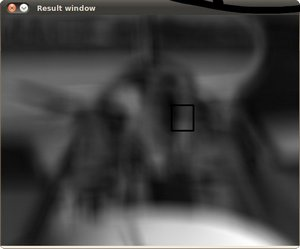Result_0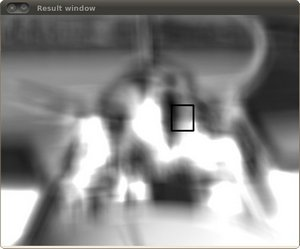Result_1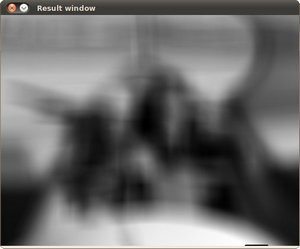Result_2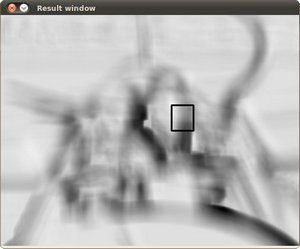Result_3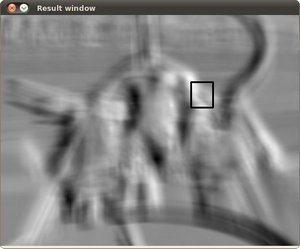Result_4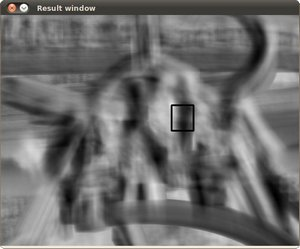Result_5
3. The right match is shown below (black rectangle around the face of the guy at the right). Notice that CCORR and CCDEFF gave erroneous best matches, however their normalized version did it right, this may be due to the fact that we are only considering the "highest match" and not the other possible high matches.